# How To Auto Sort Data By Any Column Using VBA in Microsoft Excel 2010

In this article, you will learn how to automatically sort data by a particular column.

Let us take an example:

Following is a snapshot of the data we have. Refer below snapshot: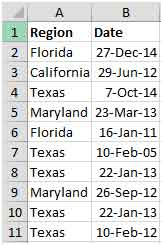We want a code that will automatically sort the data on column A as we type.

Click on Developer tab

From Code group, select Visual Basic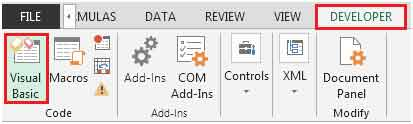Enter the following code on the sheet you are working. (sheet1 in our example)

Private Sub Worksheet_Change(ByVal Target As Range)

On Error Resume Next

If Not Intersect(Target, Range("A:A")) Is Nothing Then

Range("A1").Sort Key1:=Range("A2"), _

OrderCustom:=1, MatchCase:=False, _

Orientation:=xlTopToBottom

End If

End Sub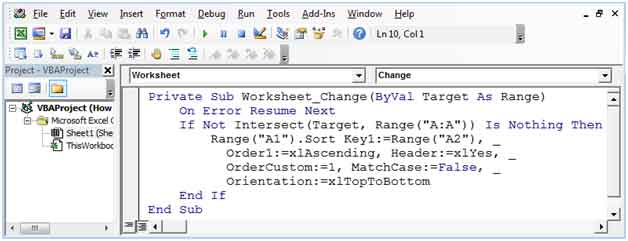Following is the result of the above code.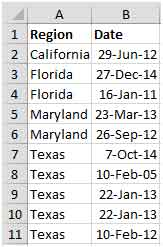The above code will activate whenever there is change in column A, if you want to activate the macro when there is any change in column B, then you have to make slight adjustment

Private Sub Worksheet_Change(ByVal Target As Range)

On Error Resume Next

If Not Intersect(Target, Range("B:B")) Is Nothing Then

Range("B1").Sort Key1:=Range("B2"), _

OrderCustom:=1, MatchCase:=False, _

Orientation:=xlTopToBottom

End If

End Sub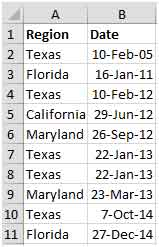In this way, you can easily sort the data by any specific column. The code will get triggered & sort the data automatically as you type information in column A (in first example) & column B (in second example). This will save a lot of time if we have to sort the data on a regular basis.

1.2.